This is “Applications of Linear Systems”, section 4.4 from the book Beginning Algebra (v. 1.0). For details on it (including licensing), click here.

Has this book helped you? Consider passing it on:
Creative Commons supports free culture from music to education. Their licenses helped make this book available to you.
DonorsChoose.org helps people like you help teachers fund their classroom projects, from art supplies to books to calculators.

## 4.4 Applications of Linear Systems

### Learning Objectives

1. Set up and solve applications involving relationships between numbers.
2. Set up and solve applications involving interest and money.
3. Set up and solve mixture problems.
4. Set up and solve uniform motion problems (distance problems).

## Problems Involving Relationships between Real Numbers

We now have the techniques needed to solve linear systems. For this reason, we are no longer limited to using one variable when setting up equations that model applications. If we translate an application to a mathematical setup using two variables, then we need to form a linear system with two equations.

Example 1: The sum of two numbers is 40 and their difference is 8. Find the numbers.

Solution:

Identify variables.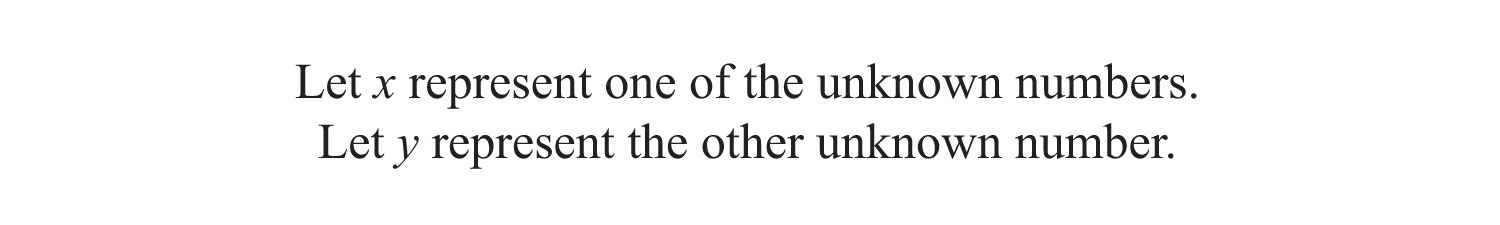Set up equations: When using two variables, we need to set up two equations. The first key phrase, “the sum of the two numbers is 40,” translates as follows: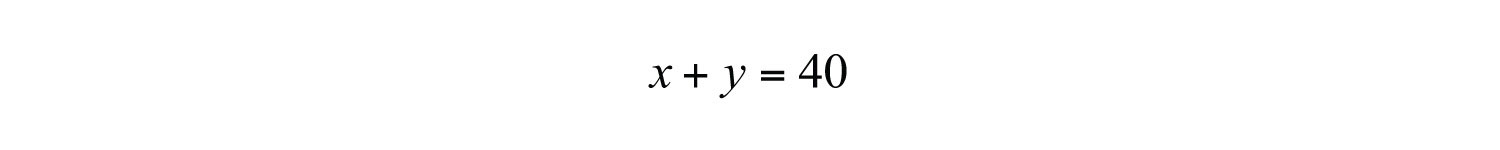And the second key phrase, “the difference is 8,” leads us to the second equation: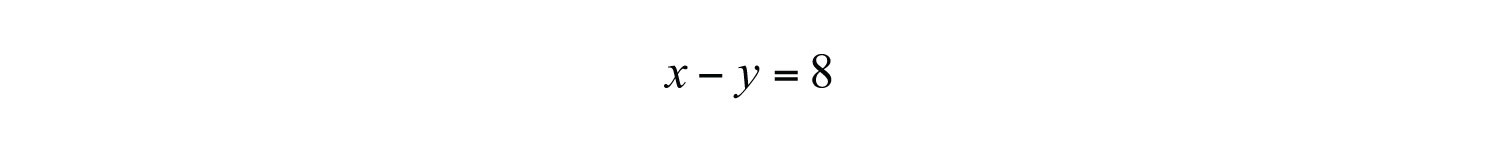Therefore, our algebraic setup consists of the following system: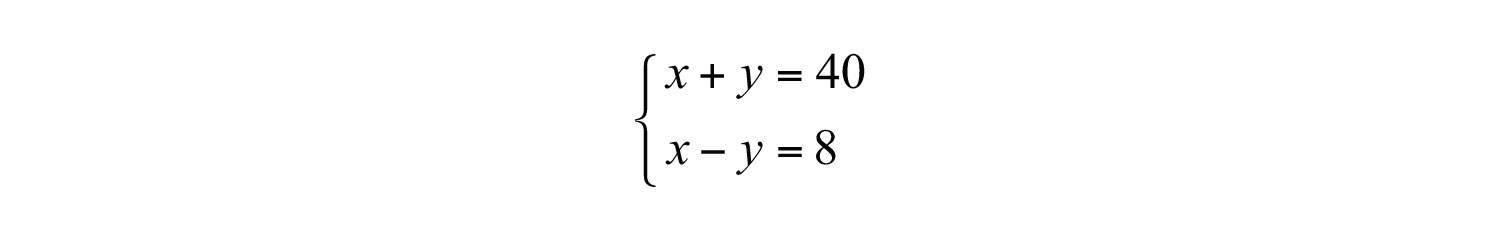Solve: We can solve the resulting system using any method of our choosing. Here we choose to solve by elimination. Adding the equations together eliminates the variable y.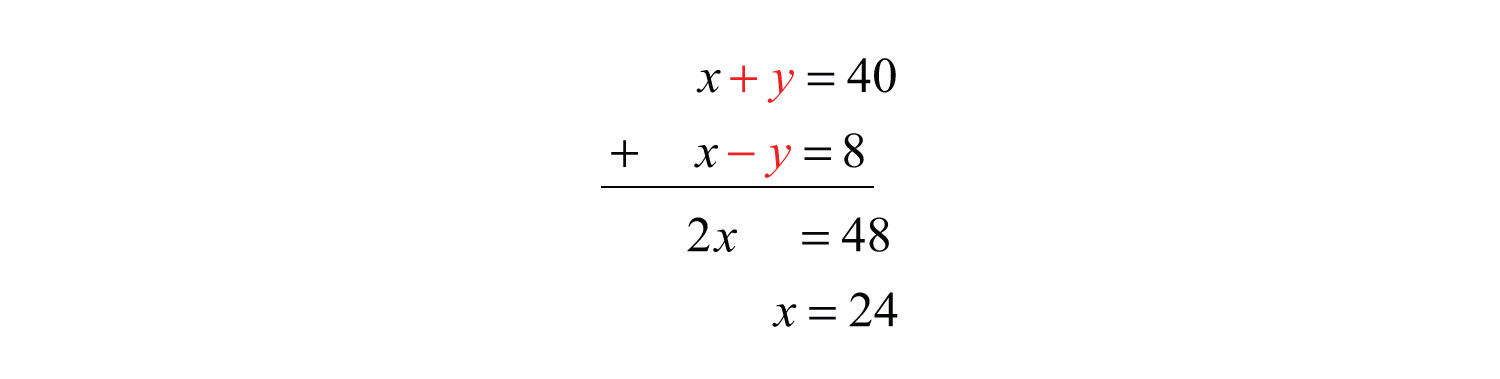Once we have x, back substitute to find y.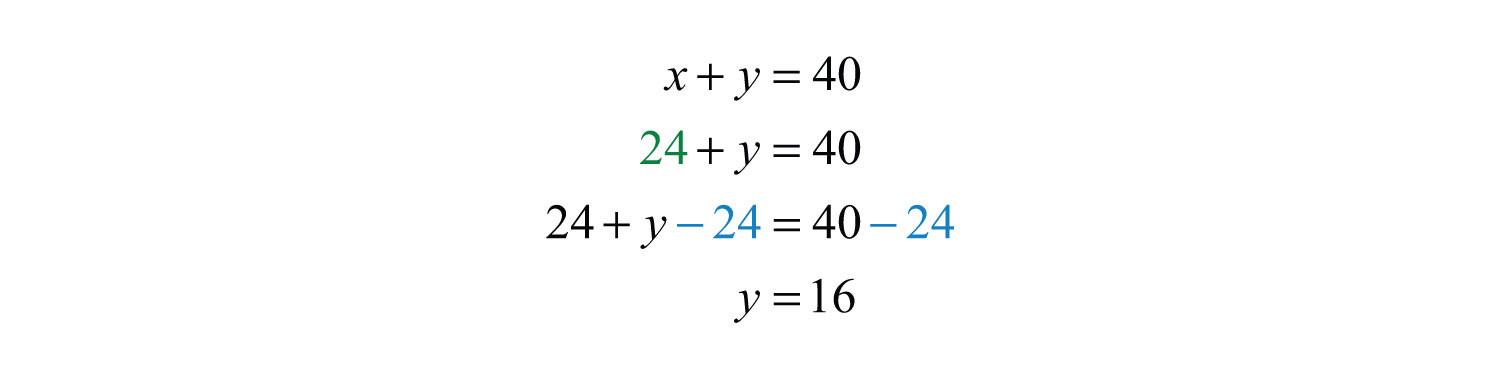Check: The sum of the two numbers should be 42 and their difference 8.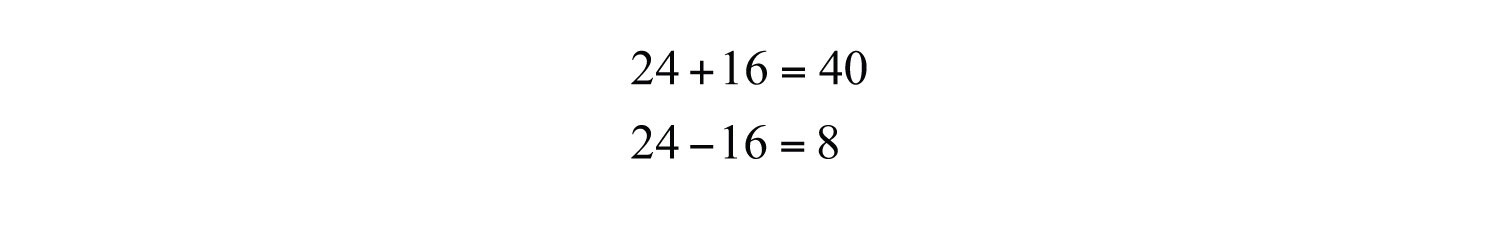Answer: The two numbers are 24 and 16.

Example 2: The sum of 9 times a larger number and twice a smaller is 6. The difference of 3 times the larger and the smaller is 7. Find the numbers.

Solution: Begin by assigning variables to the larger and smaller number.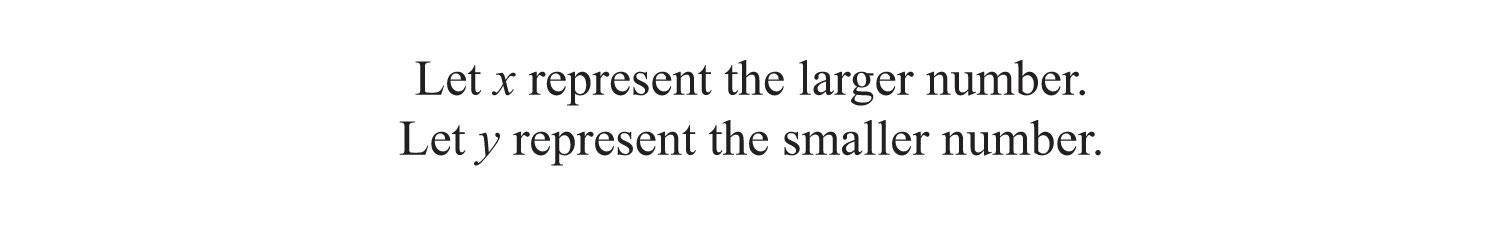The first sentence describes a sum and the second sentence describes a difference.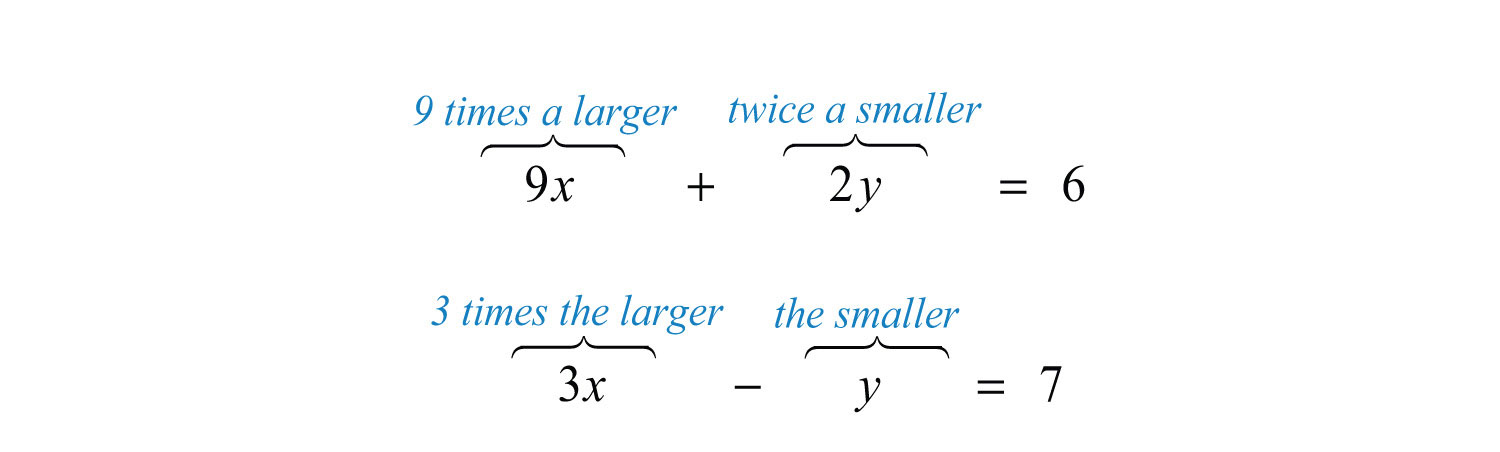This leads to the following system:Solve using the elimination method. Multiply the second equation by 2 and add.Back substitute to find y.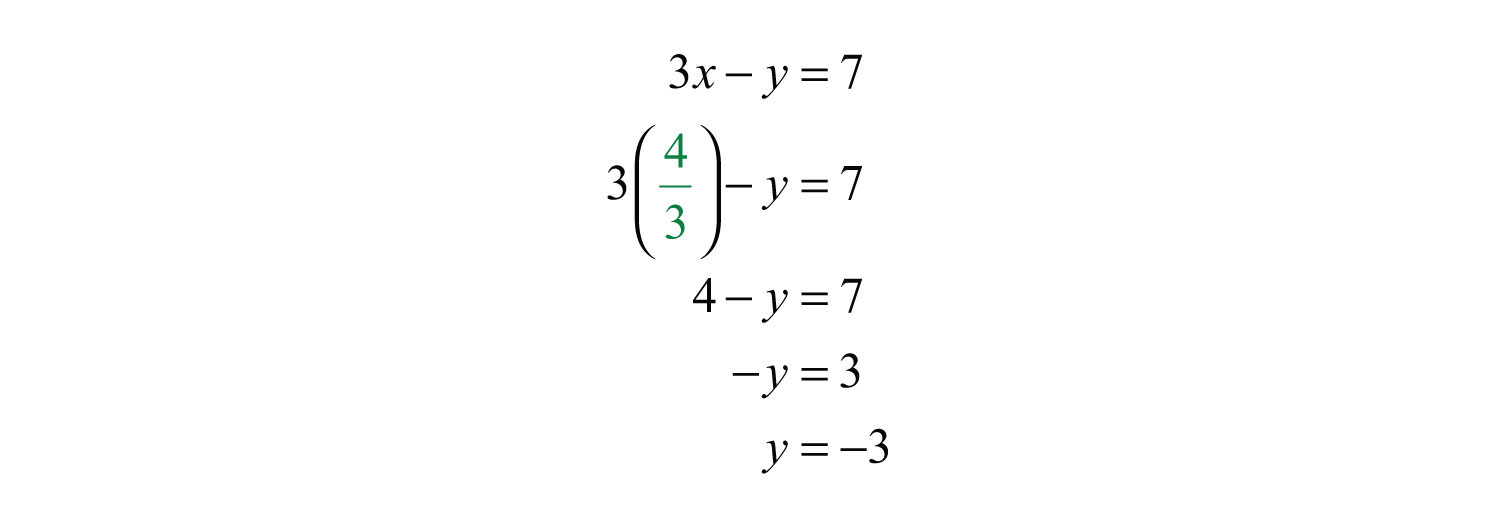Answer: The larger number is 4/3 and the smaller number is −3.

Try this! The sum of two numbers is 3. When twice the smaller number is subtracted from 6 times the larger the result is 22. Find the numbers.

Answer: The two numbers are −1/2 and 7/2.

### Video Solution

(click to see video)

## Interest and Money Problems

In this section, the interest and money problemsApplications involving simple interest and money. should seem familiar. The difference is that we will be making use of two variables when setting up the algebraic equations.

Example 3: A roll of 32 bills contains only $5 bills and$10 bills. If the value of the roll is $220, then how many of each bill are in the roll? Solution: Begin by identifying the variables.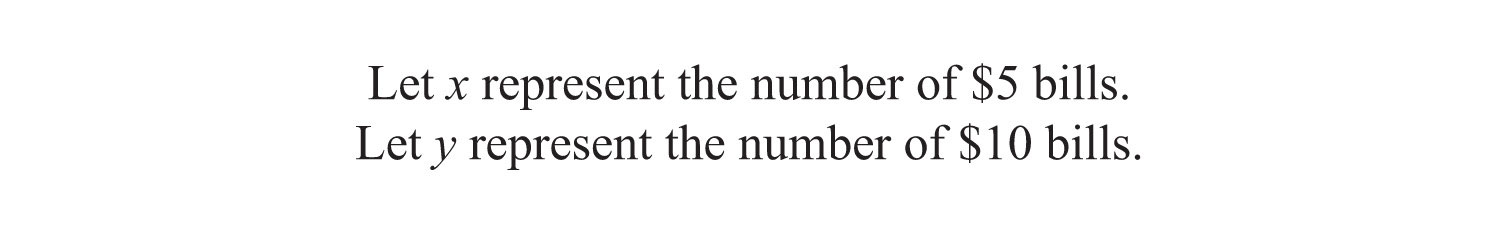When using two variables, we need to set up two equations. The first equation is created from the fact that there are 32 bills.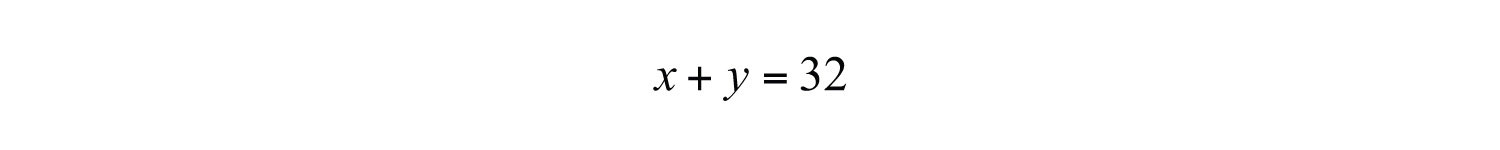The second equation sums the value of each bill: the total value is$220.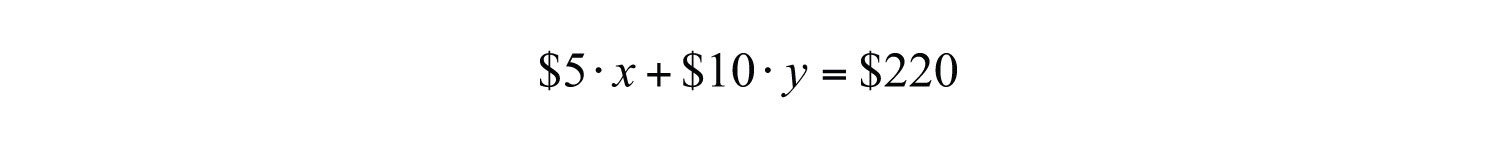Present both equations as a system; this is our algebraic setup.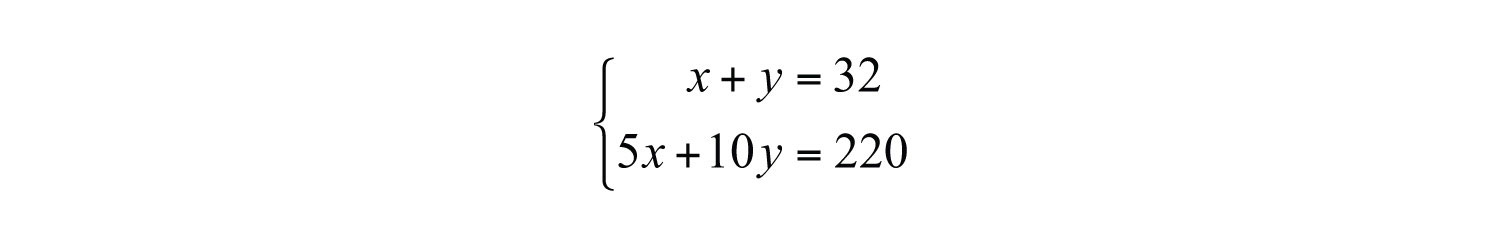Here we choose to solve by elimination, although substitution would work just as well. Eliminate x by multiplying the first equation by −5.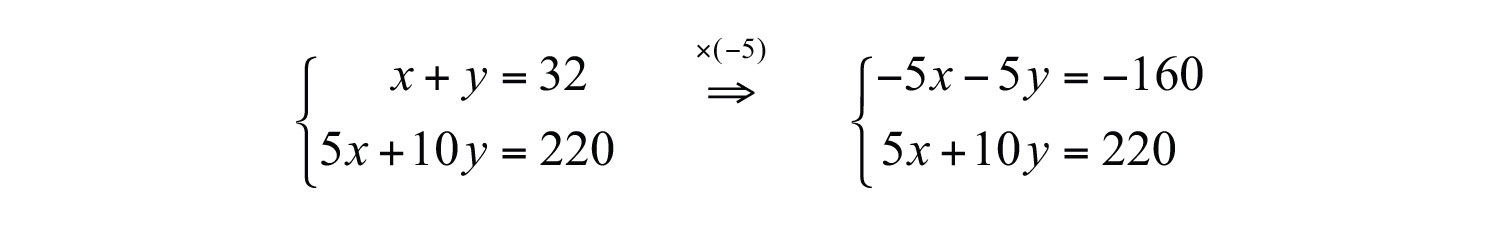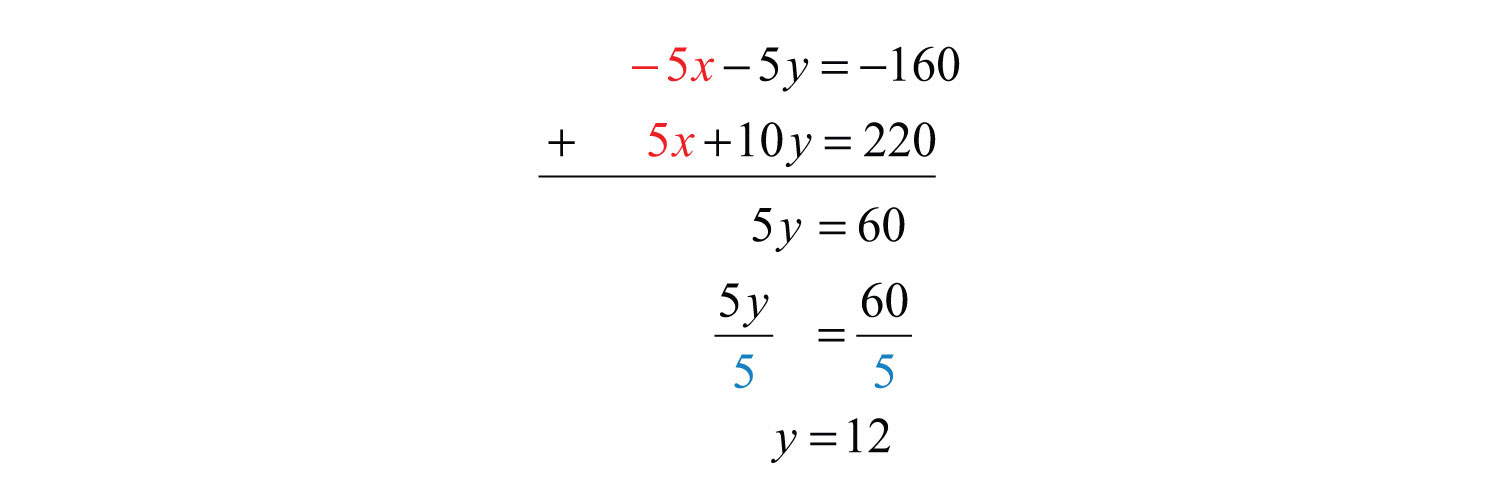Once we have y, the number of $10 bills, back substitute to find x.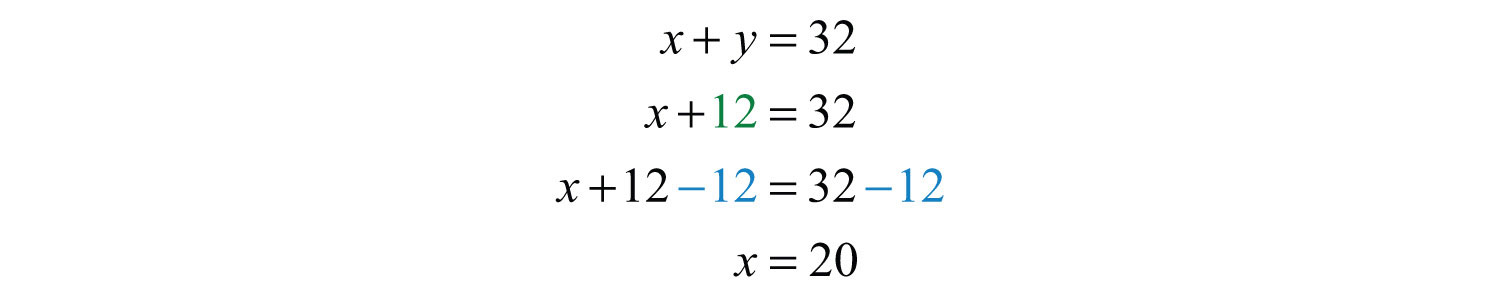Answer: There are twenty$5 bills and twelve $10 bills. The check is left to the reader. Example 4: A total of$6,300 was invested in two accounts. Part was invested in a CD at a $412%$ annual interest rate and part was invested in a money market fund at a $334%$ annual interest rate. If the total simple interest for one year was $267.75, then how much was invested in each account? Solution: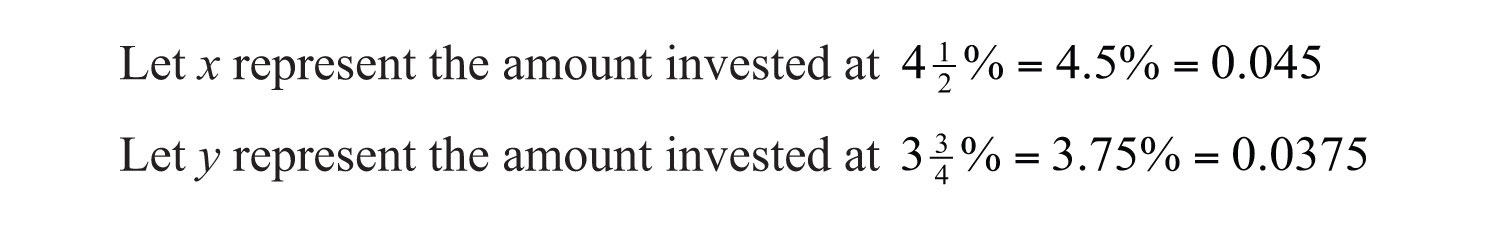The total amount in both accounts can be expressed as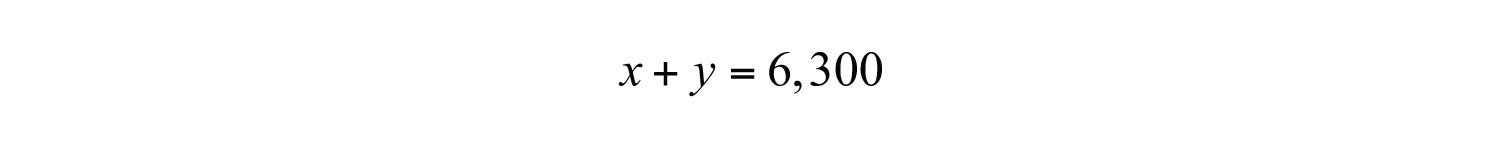To set up a second equation, use the fact that the total interest was$267.75. Recall that the interest for one year is the interest rate times the principal ($I=prt=pr⋅1=pr$). Use this to add the interest in both accounts. Be sure to use the decimal equivalents for the interest rates given as percentages.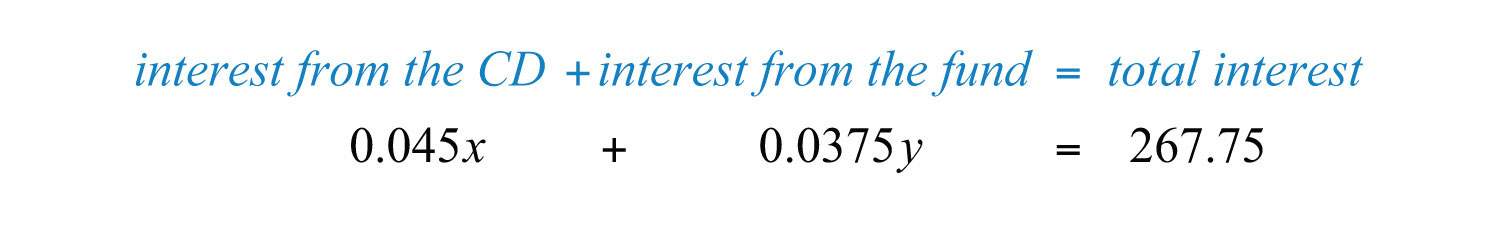These two equations together form the following linear system: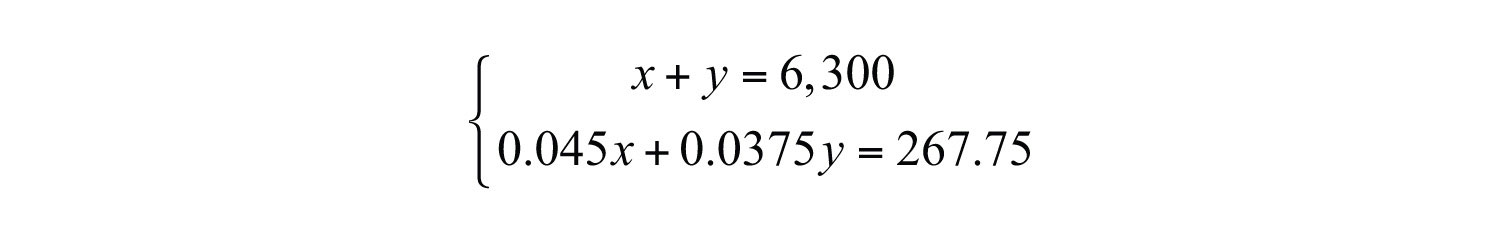Eliminate y by multiplying the first equation by −0.0375.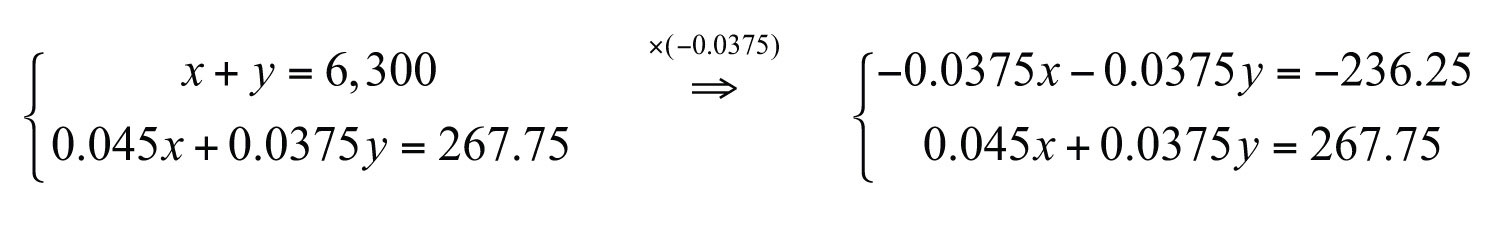Next, add the equations together to eliminate the variable y.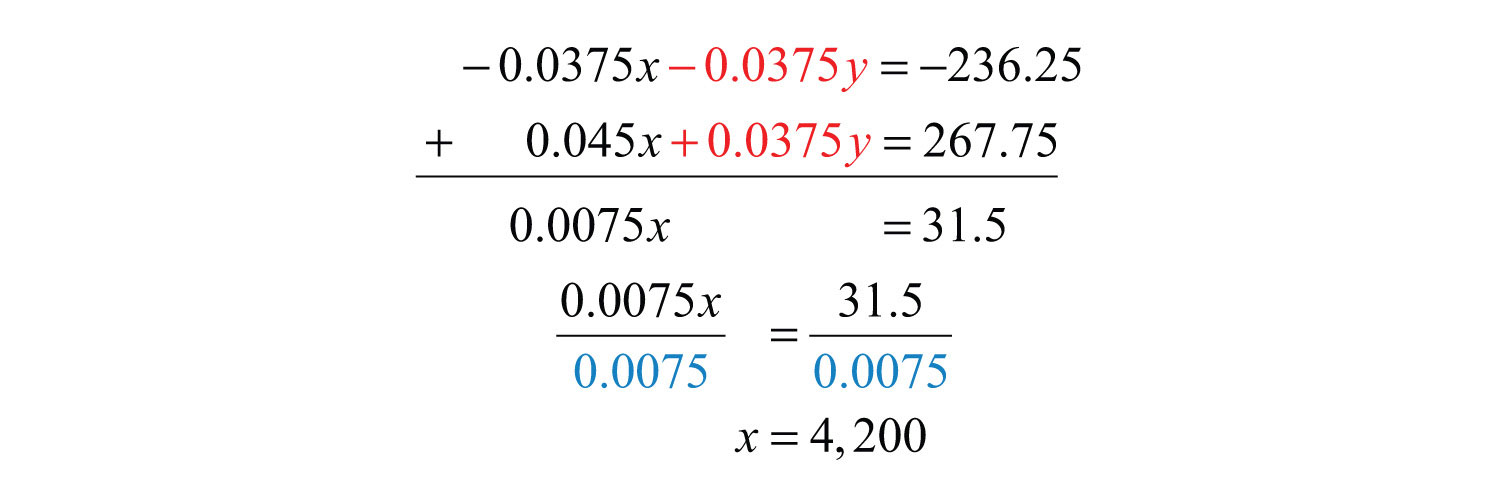Back substitute.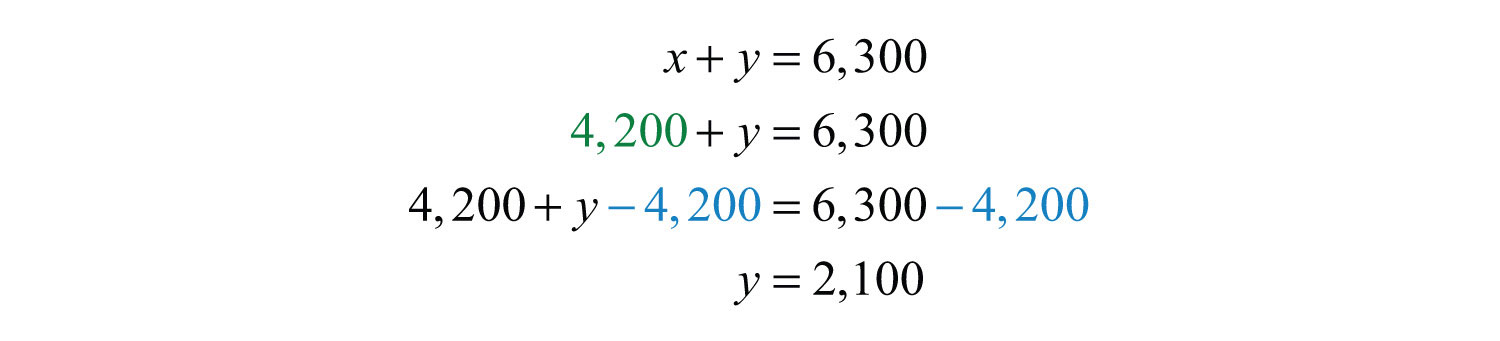Answer: $4,200 was invested at $412%$ and$2,100 was invested at $334%$.

At this point, we should be able to solve these types of problems in two ways: with one variable and now with two variables. Setting up word problems with two variables often simplifies the entire process, particularly when the relationships between the variables are not so clear.

Try this! On the first day of a two-day meeting, 10 coffees and 10 doughnuts were purchased for a total of $20.00. Since nobody drank the coffee and all the doughnuts were eaten, the next day only 2 coffees and 14 doughnuts were purchased for a total of$13.00. How much did each coffee and each doughnut cost?

Answer: Coffee: $1.25; doughnut:$0.75

### Video Solution

(click to see video)

## Mixture Problems

Mixture problemsApplications involving a mixture of amounts usually given as a percentage of some total. often include a percentage and some total amount. It is important to make a distinction between these two types of quantities. For example, if a problem states that a 20-ounce container is filled with a 2% saline (salt) solution, then this means that the container is filled with a mixture of salt and water as follows:

Percentage Amount
Salt 2% = 0.02 0.02(20 ounces) = 0.4 ounces
Water 98% = 0.98 0.98(20 ounces) = 19.6 ounces

In other words, we multiply the percentage times the total to get the amount of each part of the mixture.

Example 5: A 2% saline solution is to be combined and mixed with a 5% saline solution to produce 72 ounces of a 2.5% saline solution. How much of each is needed?

Solution: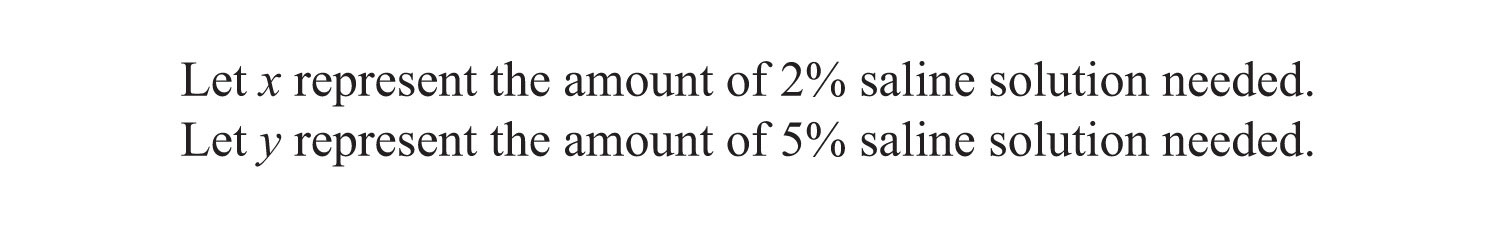The total amount of saline solution needed is 72 ounces. This leads to one equation,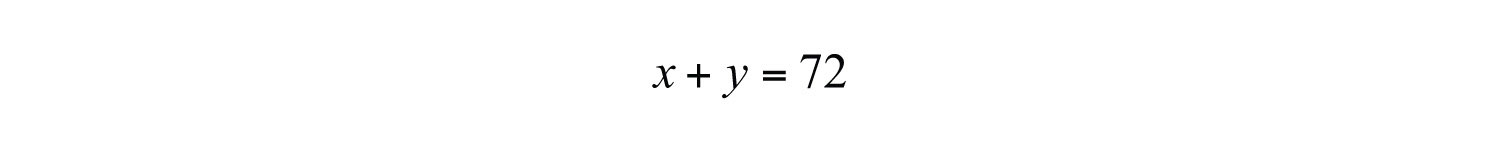The second equation adds up the amount of salt in the correct percentages. The amount of salt is obtained by multiplying the percentage times the amount, where the variables x and y represent the amounts of the solutions.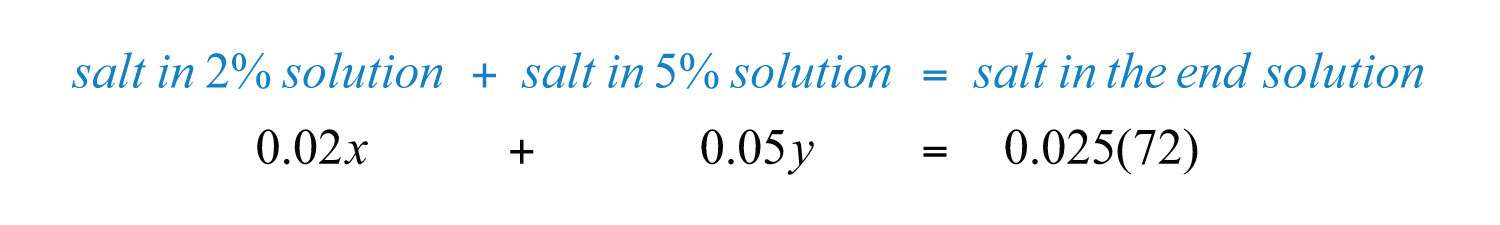The algebraic setup consists of both equations presented as a system: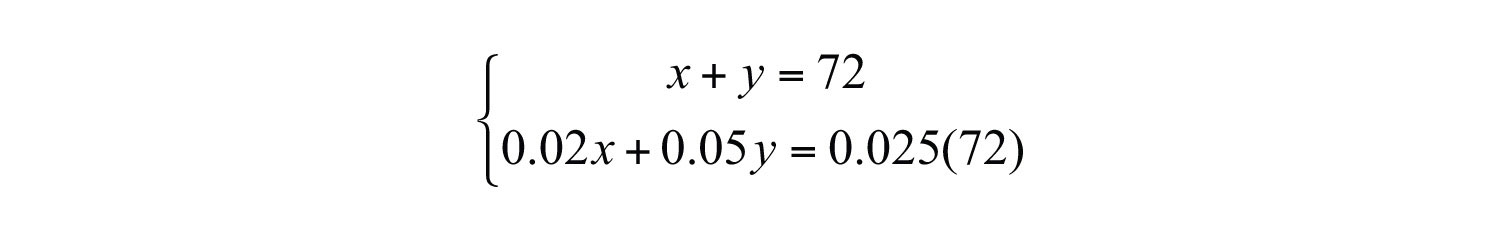Solve.Back substitute.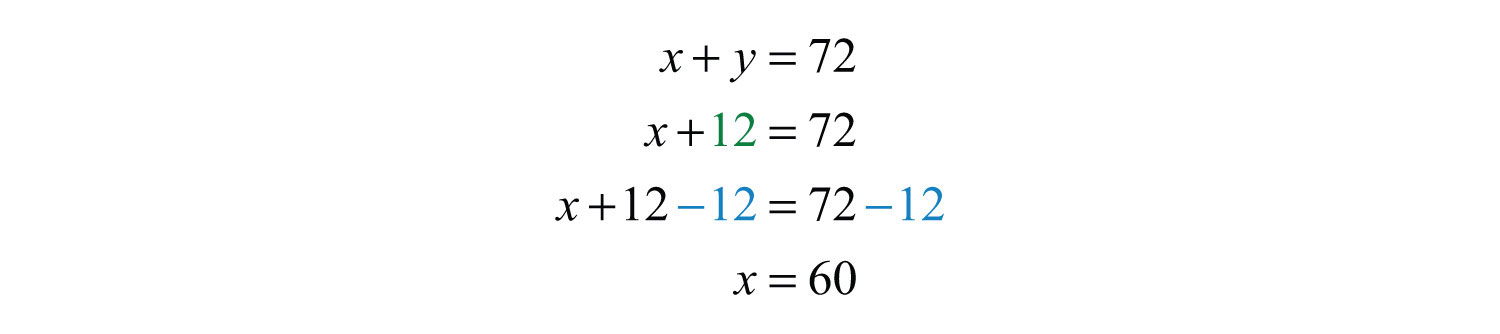Answer: We need 60 ounces of the 2% saline solution and 12 ounces of the 5% saline solution.

Example 6: A 50% alcohol solution is to be mixed with a 10% alcohol solution to create an 8-ounce mixture of a 32% alcohol solution. How much of each is needed?

Solution: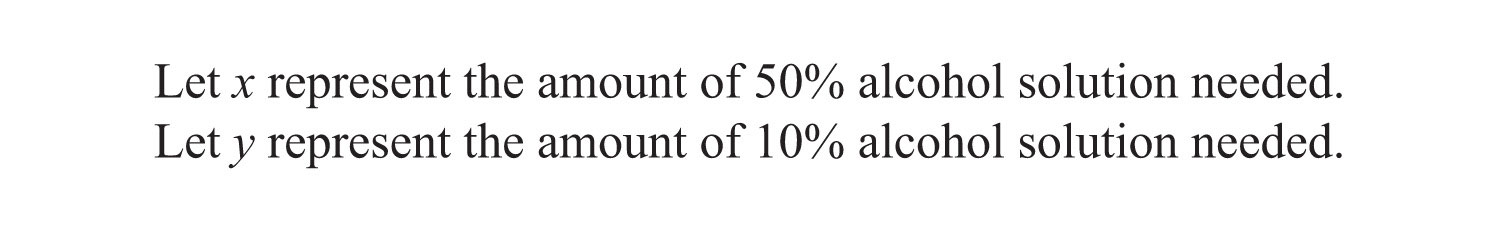The total amount of the mixture must be 8 ounces.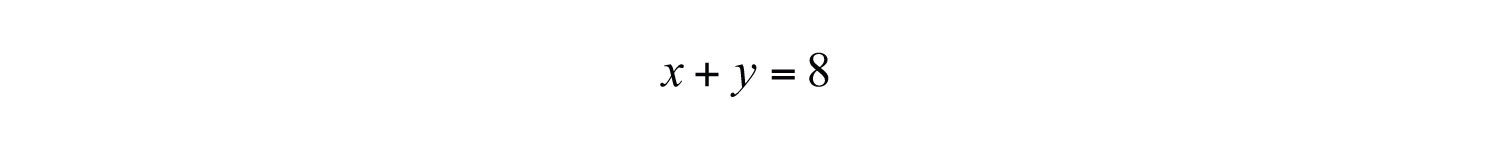The second equation adds up the amount of alcohol from each solution in the correct percentages. The amount of alcohol in the end result is 32% of 8 ounces, or 0.032(8).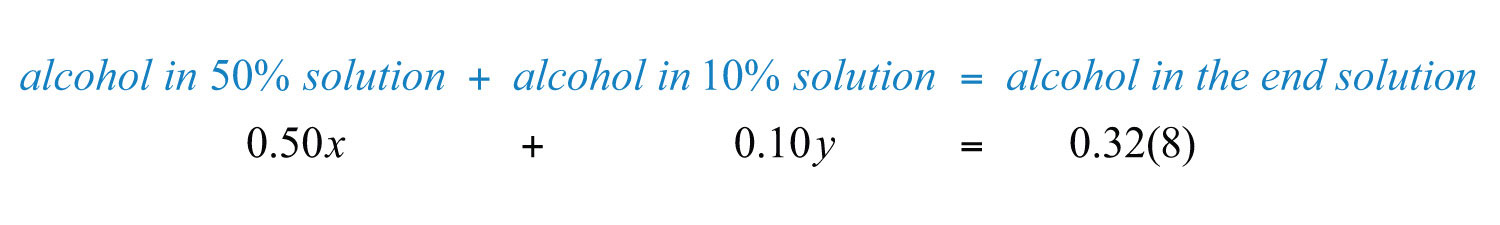Now we can form a system of two linear equations and two variables as follows: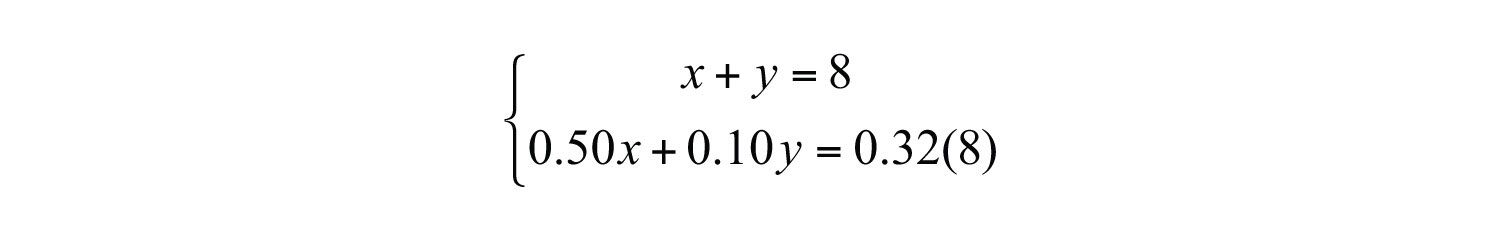In this example, multiply the second equation by 100 to eliminate the decimals. In addition, multiply the first equation by −10 to line up the variable y to eliminate.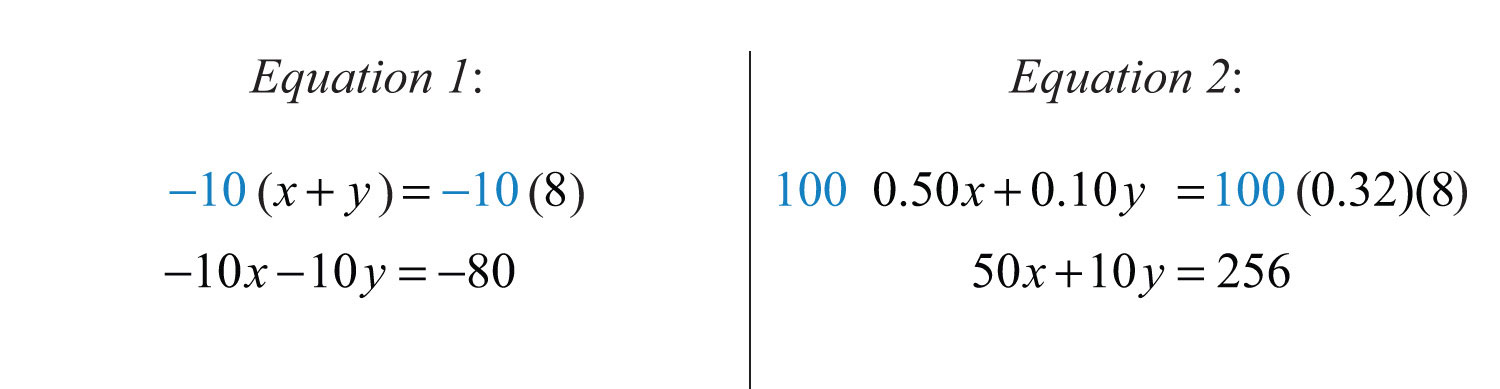We obtain the following equivalent system: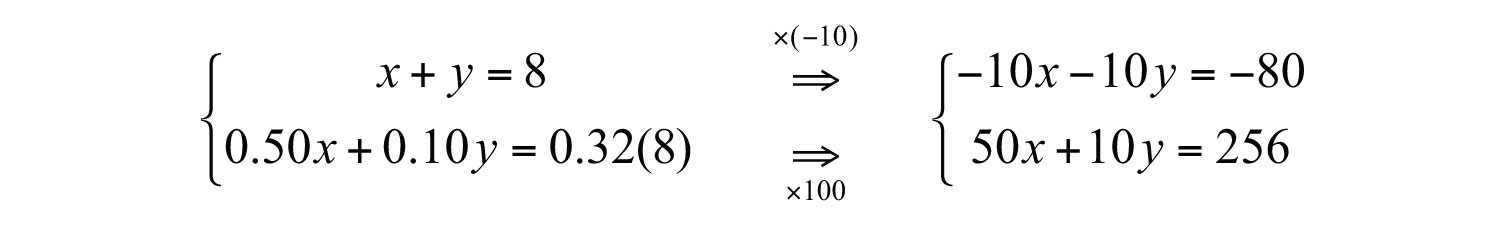Add the equations and then solve for x: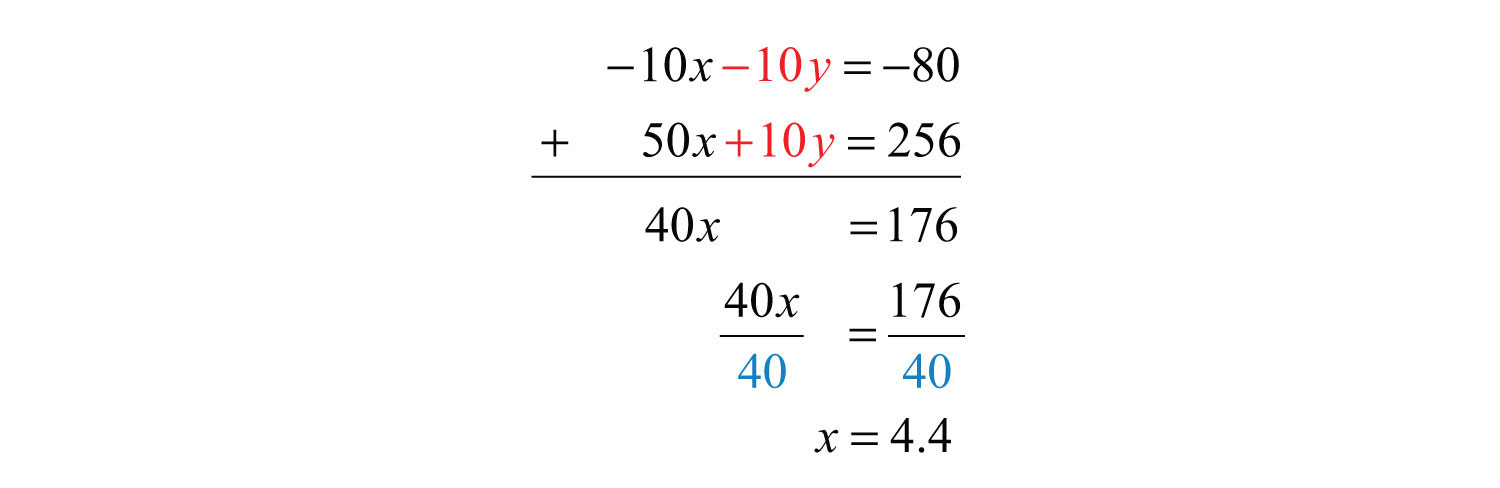Back substitute.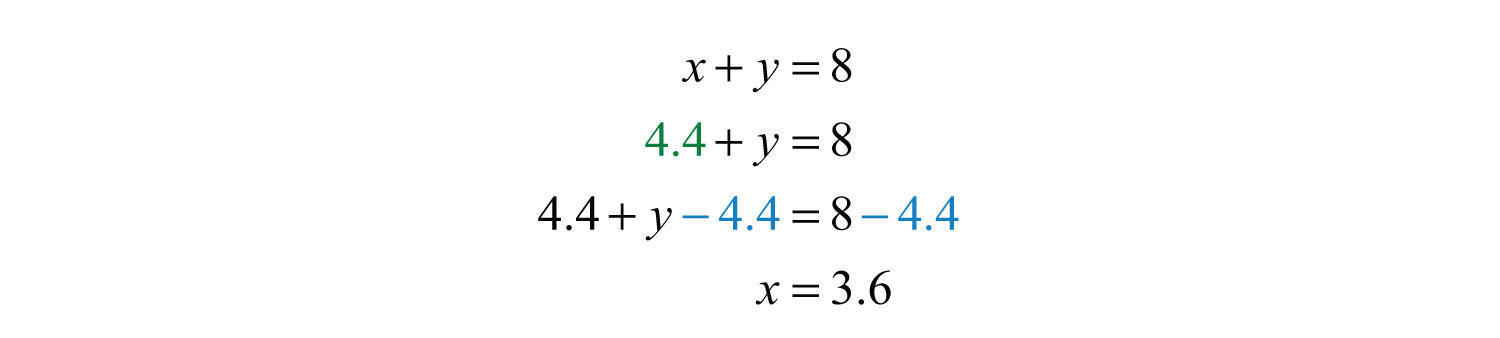Answer: To obtain 8 ounces of a 32% alcohol mixture we need to mix 4.4 ounces of the 50% alcohol solution and 3.6 ounces of the 10% solution.

Try this! A 70% antifreeze concentrate is to be mixed with water to produce a 5-gallon mixture containing 28% antifreeze. How much water and antifreeze concentrate is needed?

Answer: We need to mix 3 gallons of water with 2 gallons of antifreeze concentrate.

### Video Solution

(click to see video)

## Uniform Motion Problems (Distance Problems)

Recall that the distance traveled is equal to the average rate times the time traveled at that rate, $D=r⋅t$. These uniform motion problemsApplications relating distance, average rate, and time. usually have a lot of data, so it helps to first organize that data in a chart and then set up a linear system. In this section, you are encouraged to use two variables.

Example 7: An executive traveled a total of 8 hours and 1,930 miles by car and by plane. Driving to the airport by car, she averaged 60 miles per hour. In the air, the plane averaged 350 miles per hour. How long did it take her to drive to the airport?

Solution: We are asked to find the time it takes her to drive to the airport; this indicates that time is the unknown quantity.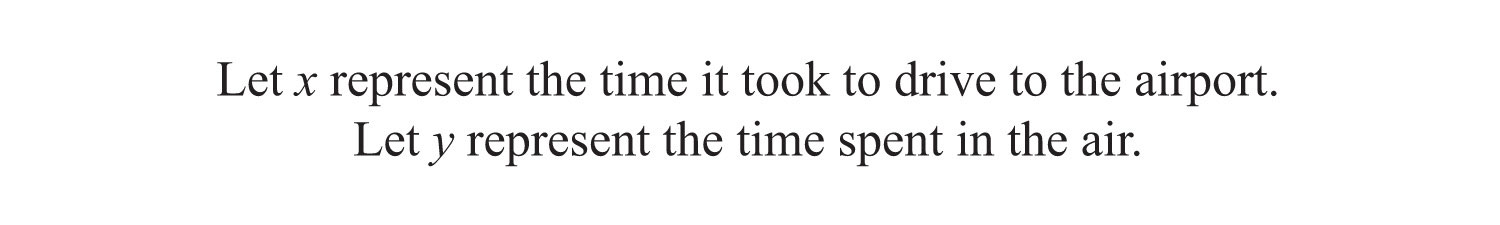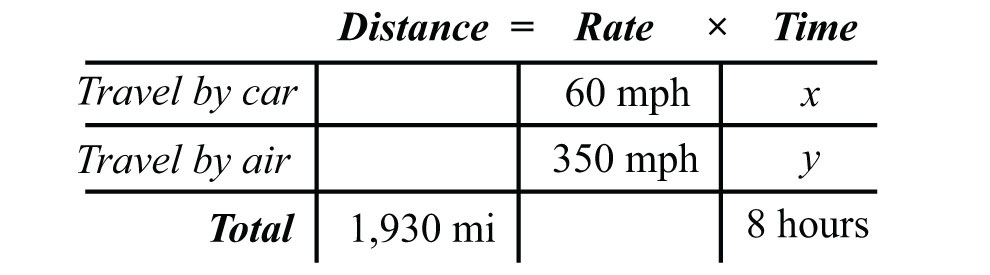Use the formula $D=r⋅t$ to fill in the unknown distances.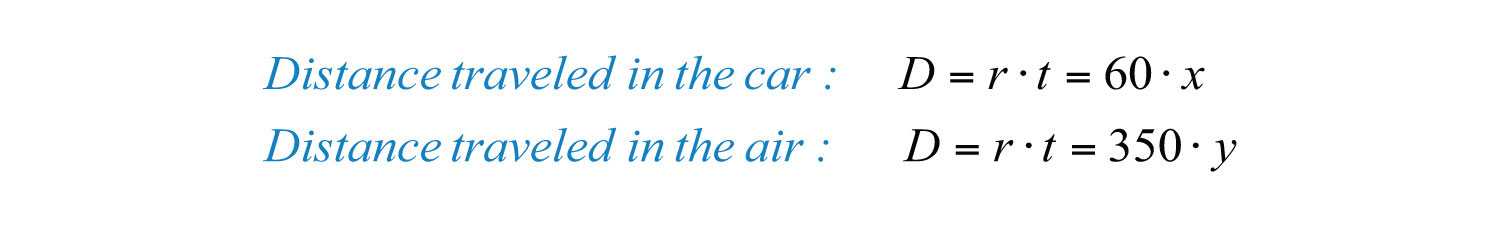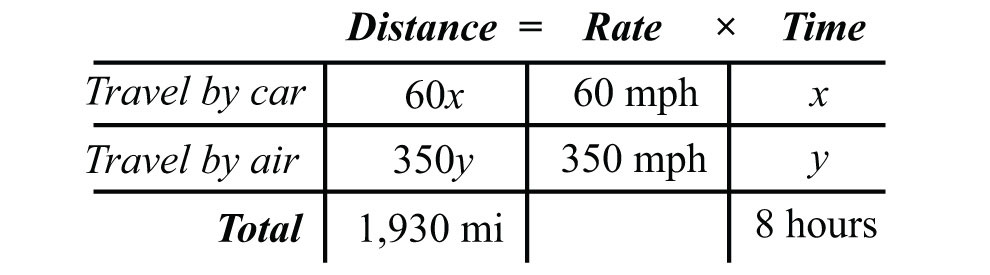The distance column and the time column of the chart help us to set up the following linear system.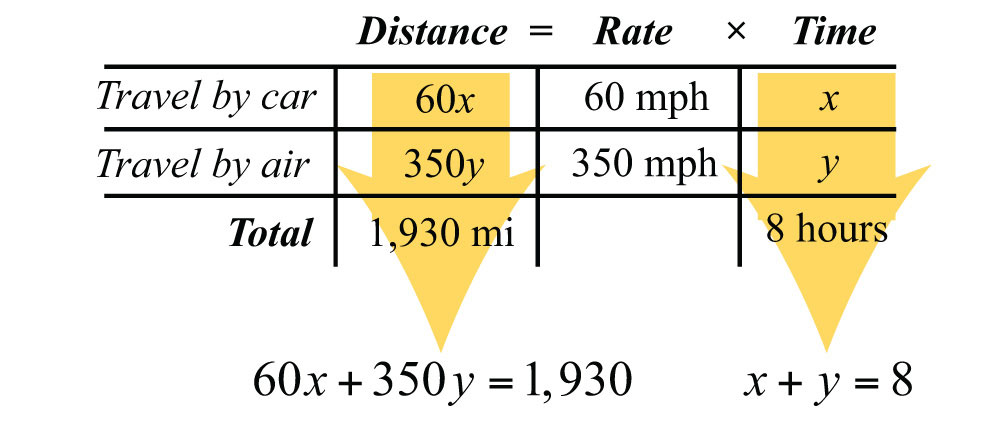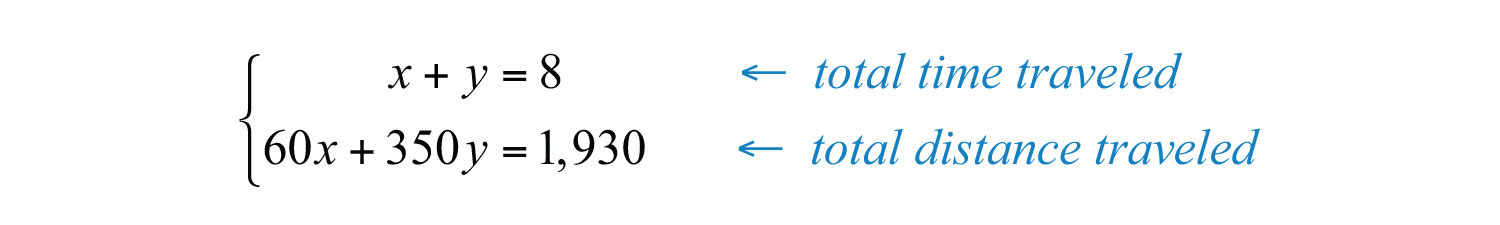Solve.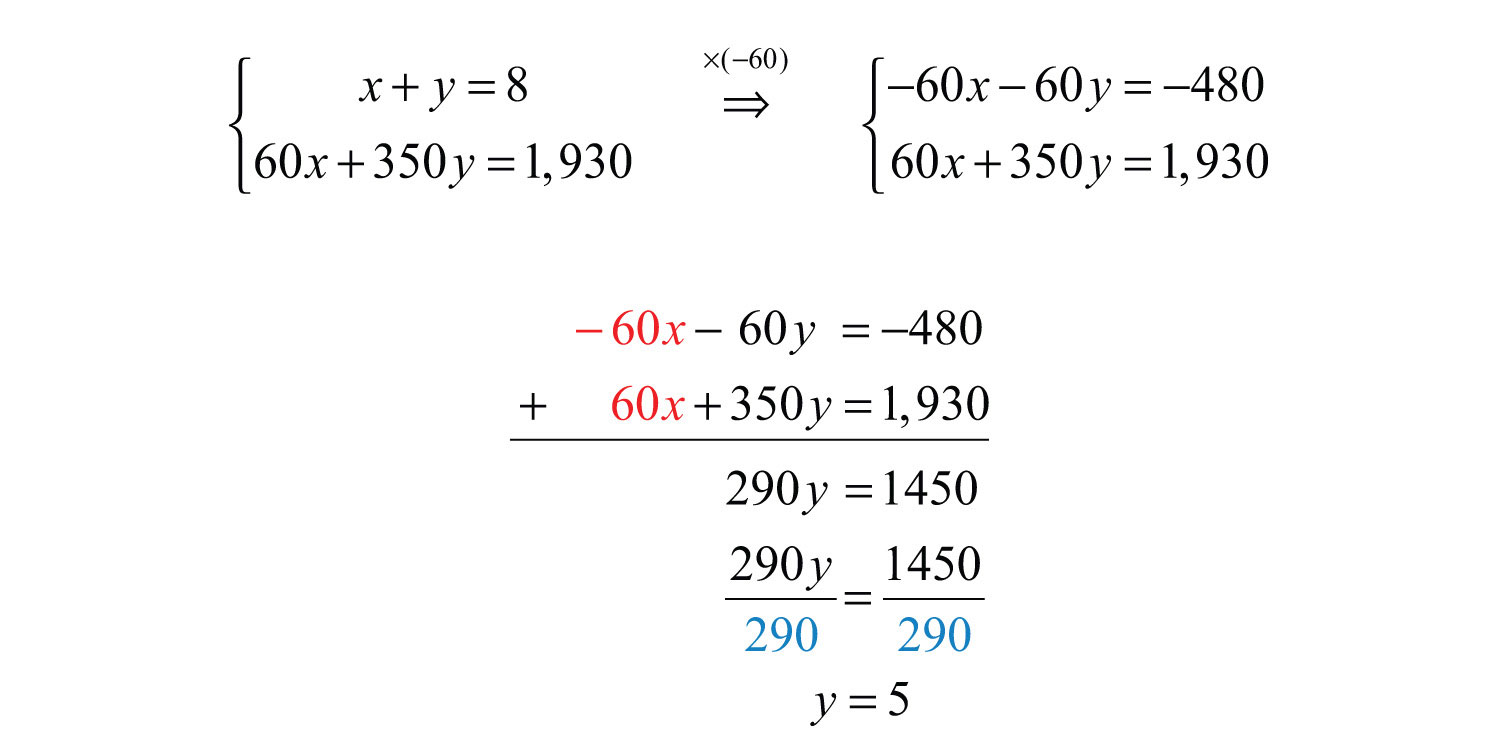Now back substitute to find the time it took to drive to the airport x: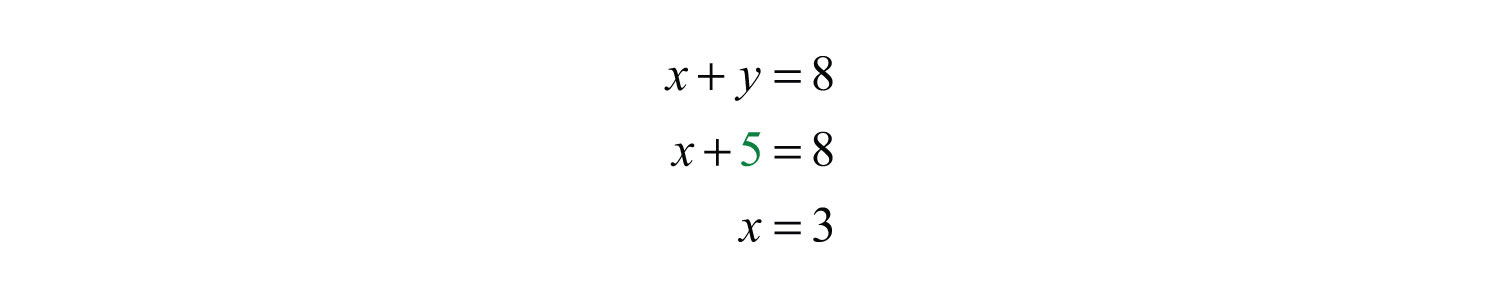Answer: It took her 3 hours to drive to the airport.

It is not always the case that time is the unknown quantity. Read the problem carefully and identify what you are asked to find; this defines your variables.

Example 8: Flying with the wind, an airplane traveled 1,365 miles in 3 hours. The plane then turned against the wind and traveled another 870 miles in 2 hours. Find the speed of the airplane and the speed of the wind.

Solution: There is no obvious relationship between the speed of the plane and the speed of the wind. For this reason, use two variables as follows: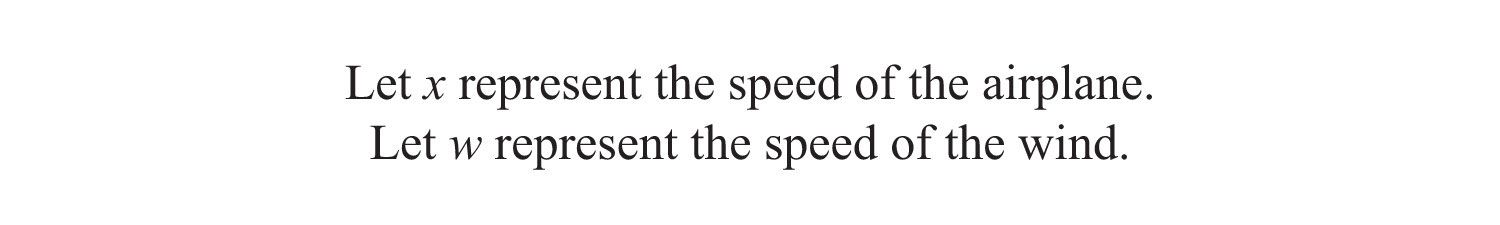Use the following chart to organize the data: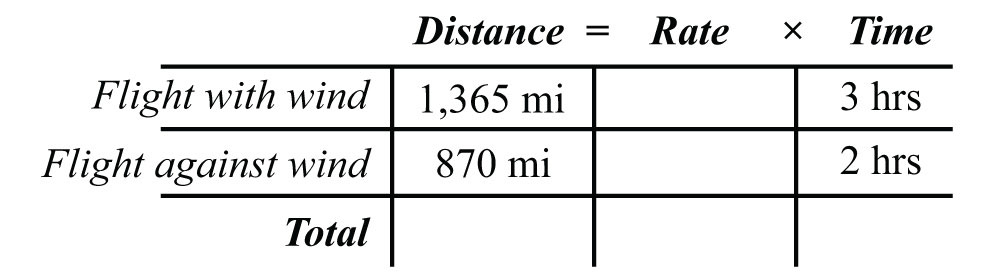With the wind, the airplane’s total speed is $x+w$. Flying against the wind, the total speed is $x−w$.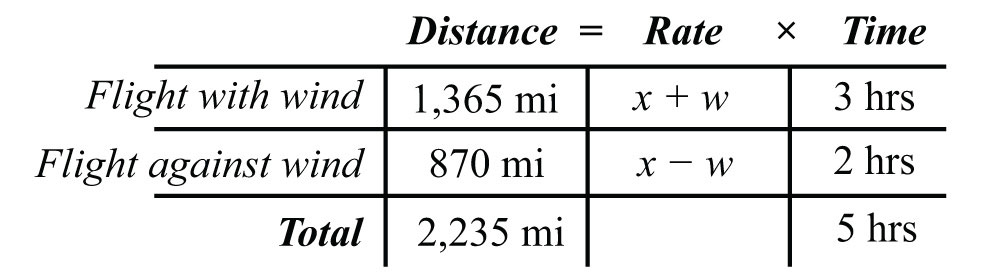Use the rows of the chart along with the formula $D=r⋅t$ to construct a linear system that models this problem. Take care to group the quantities that represent the rate in parentheses.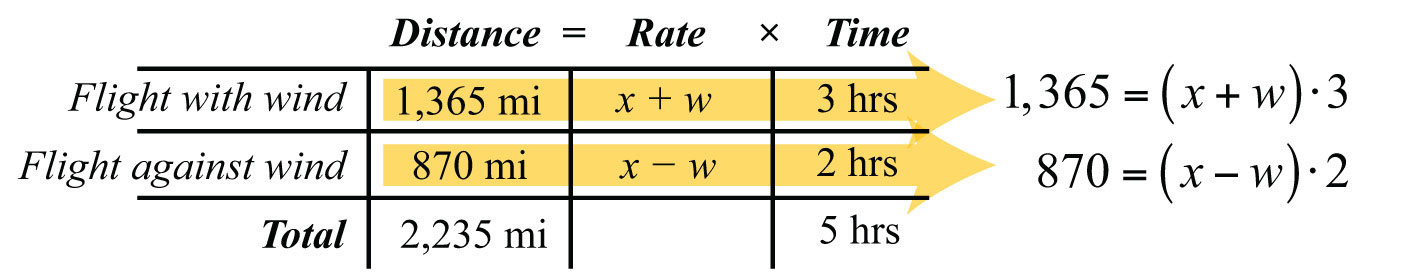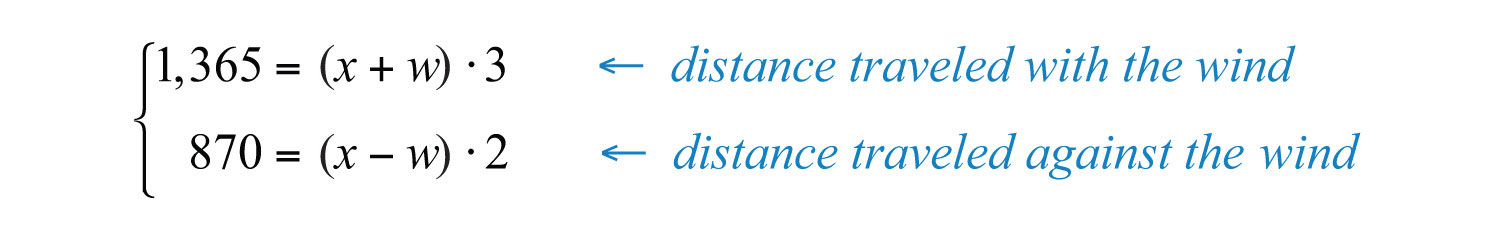If we divide both sides of the first equation by 3 and both sides of the second equation by 2, then we obtain the following equivalent system: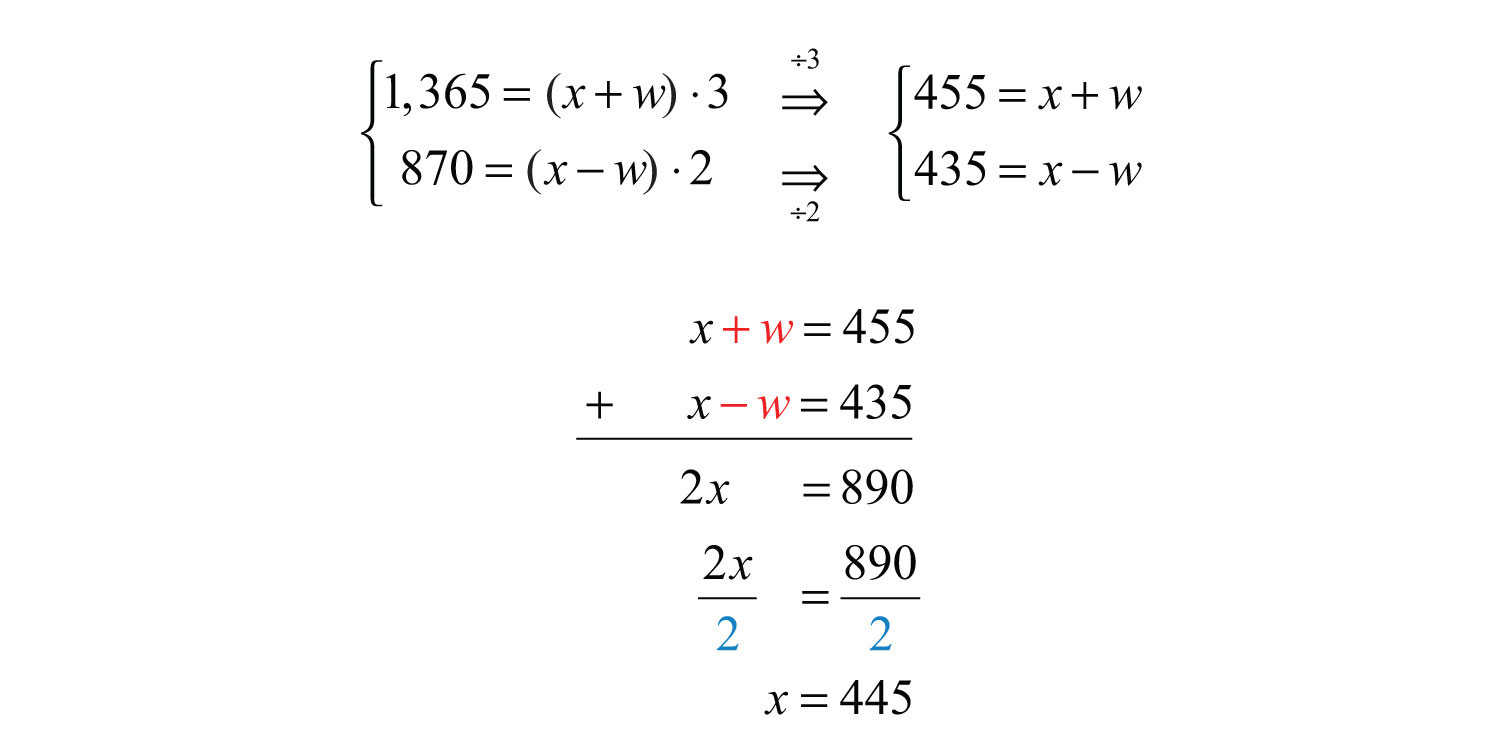Back substitute.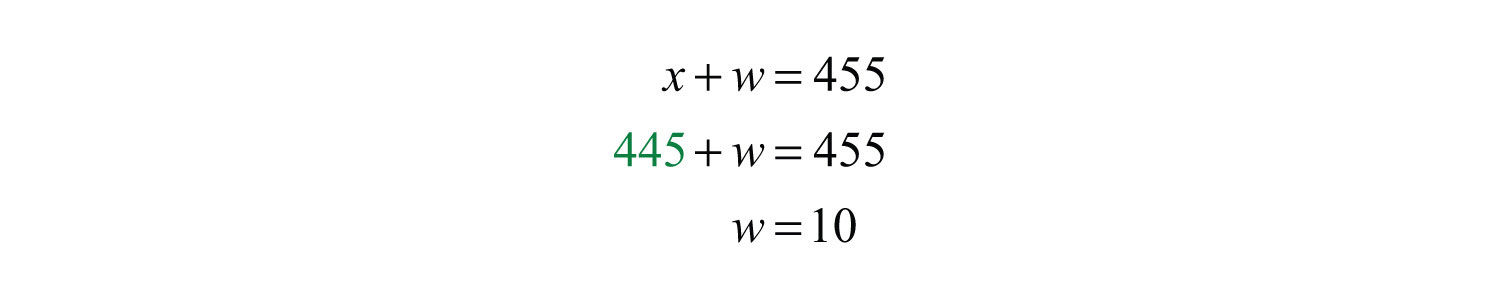Answer: The speed of the airplane is 445 miles per hour and the speed of the wind is 10 miles per hour.

Try this! A boat traveled 24 miles downstream in 2 hours. The return trip, which was against the current, took twice as long. What are the speeds of the boat and of the current?

Answer: The speed of the boat is 9 miles per hour and the speed of the current is 3 miles per hour.

### Video Solution

(click to see video)

### Key Takeaways

• Use two variables as a means to simplify the algebraic setup of applications where the relationship between unknowns is unclear.
• Carefully read the problem several times. If two variables are used, then remember that you need to set up two linear equations in order to solve the problem.
• Be sure to answer the question in sentence form and include the correct units for the answer.

### Topic Exercises

Part A: Applications Involving Numbers

Set up a linear system and solve.

1. The sum of two integers is 54 and their difference is 10. Find the integers.

2. The sum of two integers is 50 and their difference is 24. Find the integers.

3. The sum of two positive integers is 32. When the smaller integer is subtracted from twice the larger, the result is 40. Find the two integers.

4. The sum of two positive integers is 48. When twice the smaller integer is subtracted from the larger, the result is 12. Find the two integers.

5. The sum of two integers is 74. The larger is 26 more than twice the smaller. Find the two integers.

6. The sum of two integers is 45. The larger is 3 less than three times the smaller. Find the two integers.

7. The sum of two numbers is zero. When 4 times the smaller number is added to 8 times the larger, the result is 1. Find the two numbers.

8. The sum of a larger number and 4 times a smaller number is 5. When 8 times the smaller is subtracted from twice the larger, the result is −2. Find the numbers.

9. The sum of 12 times the larger number and 11 times the smaller is −36. The difference of 12 times the larger and 7 times the smaller is 36. Find the numbers.

10. The sum of 4 times the larger number and 3 times the smaller is 7. The difference of 8 times the larger and 6 times the smaller is 10. Find the numbers.

Part B: Interest and Money Problems

Set up a linear system and solve.

11. A $7,000 principal is invested in two accounts, one earning 3% interest and another earning 7% interest. If the total interest for the year is$262, then how much is invested in each account?

12. Mary has her total savings of $12,500 in two different CD accounts. One CD earns 4.4% interest and another earns 3.2% interest. If her total interest for the year is$463, then how much does she have in each CD account?

13. Sally’s $1,800 savings is in two accounts. One account earns 6% annual interest and the other earns 3%. Her total interest for the year is$93. How much does she have in each account?

14. Joe has two savings accounts totaling $4,500. One account earns $334%$ annual interest and the other earns $258%$. If his total interest for the year is$141.75, then how much is in each account?

15. Millicent has $10,000 invested in two accounts. For the year, she earns$535 more in interest from her 7% mutual fund account than she does from her 4% CD. How much does she have in each account?

16. A small business has $85,000 invested in two accounts. If the account earning 3% annual interest earns$825 more in interest than the account earning 4.5% annual interest, then how much is invested in each account?

17. Jerry earned a total of $284 in simple interest from two separate accounts. In an account earning 6% interest, Jerry invested$1,000 more than twice the amount he invested in an account earning 4%. How much did he invest in each account?

18. James earned a total of $68.25 in simple interest from two separate accounts. In an account earning 2.6% interest, James invested one-half as much as he did in the other account that earned 5.2%. How much did he invest in each account? 19. A cash register contains$10 bills and $20 bills with a total value of$340. If there are 23 bills total, then how many of each does the register contain?

20. John was able to purchase a pizza for $10.80 with quarters and dimes. If he uses 60 coins to buy the pizza, then how many of each did he have? 21. Dennis mowed his neighbor’s lawn for a jar of dimes and nickels. Upon completing the job, he counted the coins and found that there were 4 less than twice as many dimes as there were nickels. The total value of all the coins is$6.60. How many of each coin did he have?

22. Two families bought tickets for the big football game. One family ordered 2 adult tickets and 3 children’s tickets for a total of $26.00. Another family ordered 3 adult tickets and 4 children’s tickets for a total of$37.00. How much did each adult ticket cost?

23. Two friends found shirts and shorts on sale at a flea market. One bought 5 shirts and 3 shorts for a total of $51.00. The other bought 3 shirts and 7 shorts for a total of$80.00. How much was each shirt and each pair of shorts?

24. On Monday Joe bought 10 cups of coffee and 5 doughnuts for his office at a cost of $16.50. It turns out that the doughnuts were more popular than the coffee. Therefore, on Tuesday he bought 5 cups of coffee and 10 doughnuts for a total of$14.25. How much was each cup of coffee?

Part C: Mixture Problems

Set up a linear system and solve.

25. A 15% acid solution is to be mixed with a 25% acid solution to produce 12 gallons of a 20% acid solution. How much of each is needed?

26. One alcohol solution contains 12% alcohol and another contains 26% alcohol. How much of each should be mixed together to obtain 5 gallons of a 14.8% alcohol solution?

27. A nurse wishes to obtain 40 ounces of a 1.2% saline solution. How much of a 1% saline solution must she mix with a 2% saline solution to achieve the desired result?

28. A customer ordered 20 pounds of fertilizer that contains 15% nitrogen. To fill the customer’s order, how much of the stock 30% nitrogen fertilizer must be mixed with the 10% nitrogen fertilizer?

29. A customer ordered 2 pounds of a mixed peanut product containing 15% cashews. The inventory consists of only two mixes containing 10% and 30% cashews. How much of each type must be mixed to fill the order?

30. How many pounds of pure peanuts must be combined with a 20% peanut mix to produce 10 pounds of a 32% peanut mix?

31. How much cleaning fluid with 20% alcohol content, must be mixed with water to obtain a 24-ounce mixture with 10% alcohol content?

32. A chemist wishes to create a 32-ounce solution with 12% acid content. He uses two types of stock solutions, one with 30% acid content and another with 10% acid content. How much of each does he need?

33. A concentrated cleaning solution that contains 50% ammonia is mixed with another solution containing 10% ammonia. How much of each is mixed to obtain 8 ounces of a 32% ammonia cleaning formula?

34. A 50% fruit juice concentrate can be purchased wholesale. Best taste is achieved when water is mixed with the concentrate in such a way as to obtain a 12% fruit juice mixture. How much water and concentrate is needed to make a 50-ounce fruit juice drink?

35. A 75% antifreeze concentrate is to be mixed with water to obtain 6 gallons of a 25% antifreeze solution. How much water is needed?

36. Pure sugar is to be mixed with a fruit salad containing 10% sugar to produce 48 ounces of a salad containing 16% sugar. How much pure sugar is required?

Part D: Uniform Motion Problems

Set up a linear system and solve.

37. An airplane averaged 460 miles per hour on a trip with the wind behind it and 345 miles per hour on the return trip against the wind. If the total round trip took 7 hours, then how long did the airplane spend on each leg of the trip?

38. The two legs of a 330-mile trip took 5 hours. The average speed for the first leg of the trip was 70 miles per hour and the average speed for the second leg of the trip was 60 miles per hour. How long did each leg of the trip take?

39. An executive traveled 1,200 miles, part by helicopter and part by private jet. The jet averaged 320 miles per hour while the helicopter averaged 80 miles per hour. If the total trip took 4½ hours, then how long did she spend in the private jet?

40. Joe took two buses on the 463-mile trip from San Jose to San Diego. The first bus averaged 50 miles per hour and the second bus was able to average 64 miles per hour. If the total trip took 8 hours, then how long was spent in each bus?

41. Billy canoed downstream to the general store at an average rate of 9 miles per hour. His average rate canoeing back upstream was 4 miles per hour. If the total trip took 6½ hours, then how long did it take Billy to get back on the return trip?

42. Two brothers drove the 2,793 miles from Los Angeles to New York. One of the brothers, driving in the day, was able to average 70 miles per hour, and the other, driving at night, was able to average 53 miles per hour. If the total trip took 45 hours, then how many hours did each brother drive?

43. A boat traveled 24 miles downstream in 2 hours. The return trip took twice as long. What was the speed of the boat and the current?

44. A helicopter flying with the wind can travel 525 miles in 5 hours. On the return trip, against the wind, it will take 7 hours. What are the speeds of the helicopter and of the wind?

45. A boat can travel 42 miles with the current downstream in 3 hours. Returning upstream against the current, the boat can only travel 33 miles in 3 hours. Find the speed of the current.

46. A light aircraft flying with the wind can travel 180 miles in 1½ hours. The aircraft can fly the same distance against the wind in 2 hours. Find the speed of the wind.

Part E: Discussion Board

47. Compose a number or money problem that can be solved with a system of equations of your own and share it on the discussion board.

48. Compose a mixture problem that can be solved with a system of equations of your own and share it on the discussion board.

49. Compose a uniform motion problem that can be solved with a system of equations of your own and share it on the discussion board.

1: The integers are 22 and 32.

3: The integers are 8 and 24.

5: The integers are 16 and 58.

7: The two numbers are −1/4 and 1/4.

9: The smaller number is −4 and the larger is 2/3.

11: $5,700 at 3% and$1,300 at 7%

13: $1,300 at 6% and$500 at 3%

15: $8,500 at 7% and$1,500 at 4%

17: $1,400 at 4% and$3,800 at 6%

19: 12 tens and 11 twenties

21: 52 dimes and 28 nickels

23: Shirts: $4.50; shorts:$9.50

25: 6 gallons of each

27: 32 ounces of the 1% saline solution and 8 ounces of the 2% saline solution

29: 1.5 pounds of the 10% cashew mix and 0.5 pounds of the 30% cashew mix

31: 12 ounces of cleaning fluid

33: 4.4 ounces of the 50% ammonia solution and 3.6 ounces of the 10% ammonia solution

35: 4 gallons

37: The airplane flew 3 hours with the wind and 4 hours against the wind.

39: 3.5 hours

41: 4.5 hours

43: Boat: 9 miles per hour; current: 3 miles per hour

45: 1.5 miles per hour